Data Structures and Algorithms with Object-Oriented Design Patterns in C### Example-Computing Binomial Coefficients

Consider the problem of computing the binomial coefficient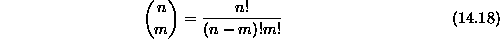given non-negative integers n and m (see Theorem).

The problem with implementing directly Equationis that the factorials grow quickly with increasing n and m. For example,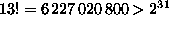. Therefore, it is not possible to represent n! for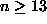using 32-bit integers. Nevertheless it is possible to represent the binomial coefficients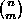up to n=33 without overflowing. For example,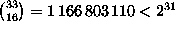.

Consider the following recursive definition of the binomial coefficients:This formulation does not require the computation of factorials. In fact, the only computation needed is addition.

If we implement Equationdirectly as a recursive method, we get a method whose running time is given by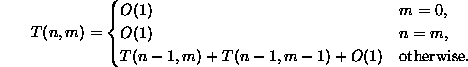which is very similar to Equation. In fact, we can show that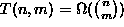which (by Equation) is not a very good running time at all! Again the problem with the direct recursive implementation is that it does far more work than is needed because it solves the same subproblem many times.

An alternative to the top-down recursive implementation is to do the calculation from the bottom up. In order to do this we compute the series of sequences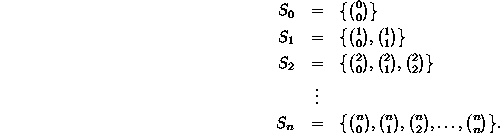Notice that we can compute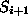from the information contained in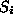simply by using Equation. Tableshows the sequence in tabular form--the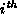row of the table corresponds the sequence. This tabular representation of the binomial coefficients is known as Pascal's triangle .n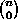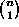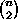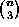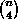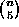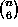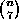0 1 1 1 1 2 1 2 1 3 1 3 3 1 4 1 4 6 4 1 5 1 5 10 10 5 1 6 1 6 15 20 15 6 1 7 1 7 21 35 35 21 7 1

Programdefines the method Binom which takes two integer arguments n and m and computes the binomial coefficientby computing Pascal's triangle. According to Equation, each subsequent row depends only on the preceding row--it is only necessary to keep track of one row of data. The implementation shown uses an array of length n to represent a row of Pascal's triangle. Consequently, instead of a table of size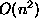, the algorithm gets by with O(n) space. The implementation has been coded carefully so that the computation can be done in place. That is, the elements ofare computed in reverse so that they can be written over the elements ofthat are no longer needed.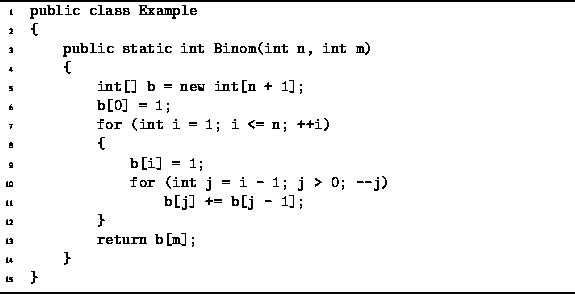Program: Dynamic programming example--computing Binomial coefficients.

The worst-case running time of the Binom method given in Programis clearly.Copyright © 2001 by Bruno R. Preiss, P.Eng. All rights reserved.